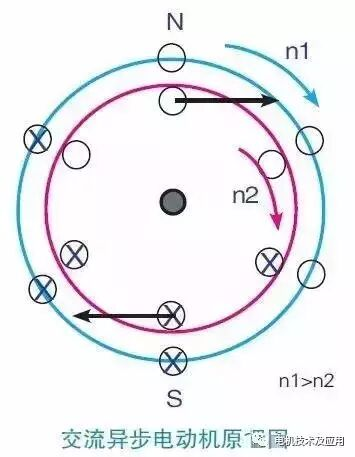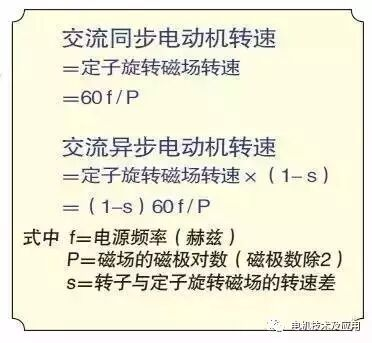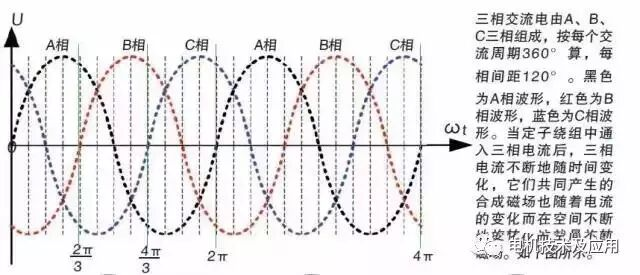Home > Enterprise News
News# Who will decide the motor speed of the electric car?

View:33802/20/2017

In order to clarify the speed of AC asynchronous motor and what factors, where the principle of induction motor will be introduced again:

First, the stator windings of the asynchronous motor are connected to the three-phase power supply. Since the current between the phase and the phase of the three-phase power supply is 120 ° out of phase and the three windings in the stator are also 120 ° apart in the spatial orientation, In this way, the stator winding will produce a rotating magnetic field.

Secondly, after the stator winding produces a rotating magnetic field, the rotor bar (cage or winding) will cut the magnetic field of the rotating magnetic field to generate the induced current, and then produce the rotor induced magnetic field. Under the action of Lenz's law, the rotor will rotate in the same direction as the rotating magnetic field of the stator, and the rotational speed of the rotor is less than 2% ~ 5% of the rotational speed of the stator rotating magnetic field, that is, the speed of the rotor is slower than that of the stator rotating magnetic field The If it is assumed that the rotational speed difference between the rotor and the stator rotating magnetic field is s, then:

Asynchronous motor speed = rotor speed = stator rotation magnetic field speed × (1-s)

Therefore, as long as the rotation speed of the stator rotating magnetic field is controlled, the rotational speed of the motor can be controlled at the same time.

Who is the speed of the permanent magnet synchronous motor?

The following are the same as the "

The rotor magnetic field of the permanent magnet synchronous motor is independent of the rotating magnetic field of the stator. It is a self-generated magnetic field by the permanent magnet embedded by the rotor. Therefore, the rotation of the rotor is not limited by the law of Lenz, but according to the principle of homosexual repulsion and opposite sex , And the rotor speed and the stator magnetic field is exactly the same (also known as the synchronous motor), that is, the rotor and stator magnetic field speed difference s = 0,

Synchronous motor speed = rotor speed = stator rotation magnetic field speed

Therefore, the permanent magnet synchronous motor and AC asynchronous motor, as long as the control of the stator rotation of the magnetic field speed, you can simultaneously control the motor speed.The speed of the motor

The following are the same as the "

As mentioned in the previous section, whether it is an AC asynchronous motor or a permanent magnet synchronous motor, it is possible to control the rotational speed of the motor as long as the rotational speed of the stator rotating magnetic field is adjusted.

The following are the same as the "

Stator rotation magnetic field speed and power frequency and magnetic pole number, the specific formula is:

Rotation speed of stator rotating magnetic field: n = 60f / P

The following are the same as the "

Where r is the stator rotation magnetic field speed (r / min); f is the power frequency (Hz); P is the magnetic field of the pole pair (the number of poles except 2).

The following are the same as the "

And then according to the formula in the previous section, you can get the following formula:

AC asynchronous motor speed: n = (1-s) 60f / P

Permanent magnet synchronous motor speed: n = 60f / P

The following are the same as the "

Where s is the difference between the rotational speed of the magnetic field and the rotational speed of the rotor (about 2% to 5%).

According to this formula we know that the control AC asynchronous motor and permanent magnet synchronous motor speed are the same, there are two ways:

1) variable magnetic pole method (ie, adjust P). The following are the same as the "

2) frequency conversion (ie, regulation f).

The following are the same as the "

In the past, the method of changing the magnetic pole is used to adjust the rotational speed of the motor. However, with the advancement of the semiconductor technology and the electronic technology, the electric vehicles are now adjusting the frequency of the power supply, that is, the frequency control, to realize the control of the AC motor speed, Stepless speed regulation.

The following are the same as the "

Frequency control in life is no stranger, we are now home air conditioning are used frequency control, are by adjusting the power frequency to adjust the temperature of air conditioning. As for the principle of what is the frequency, how to achieve frequency, and that is, electrical automation and professional electronic content, and do not repeat them here.No Comment
##### I want to comment
Content *
Verification Code*Click Refresh